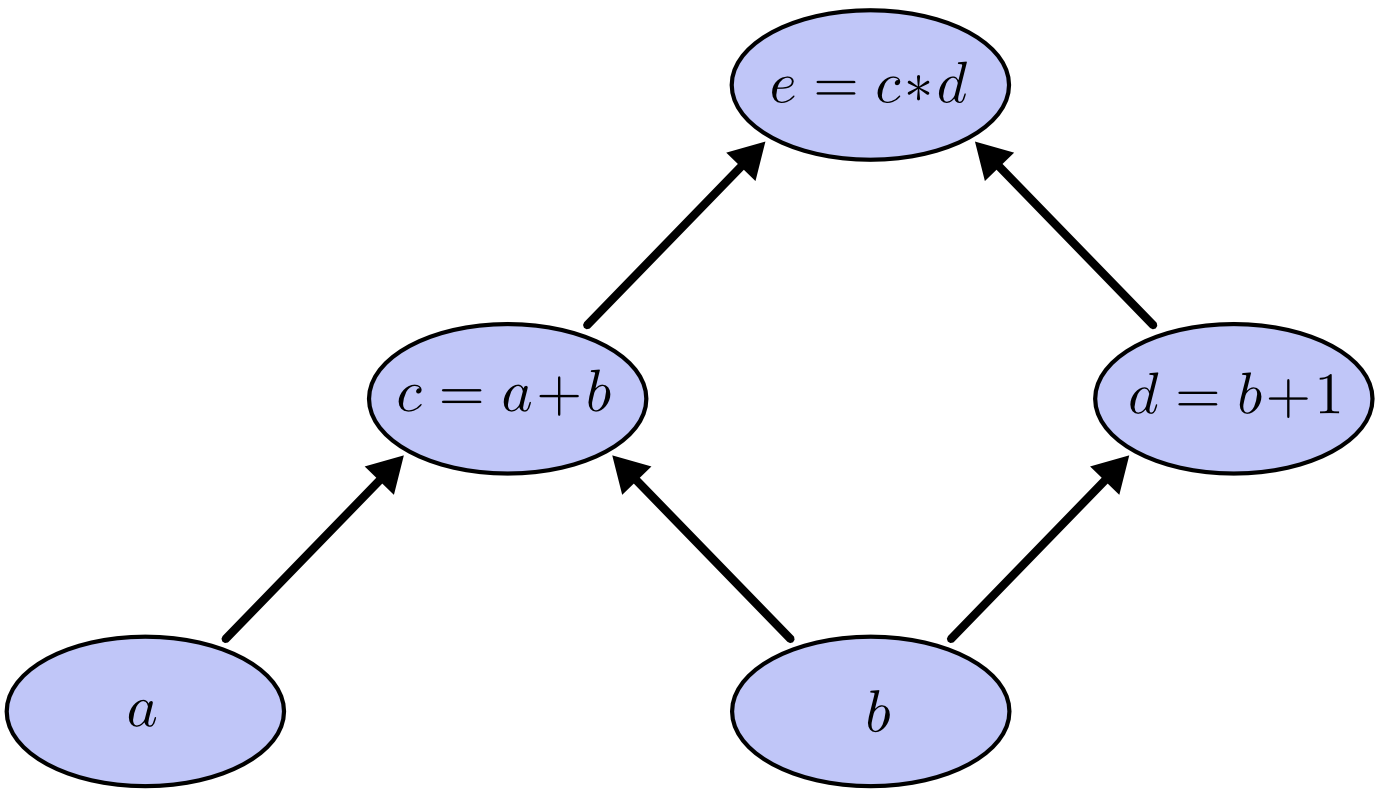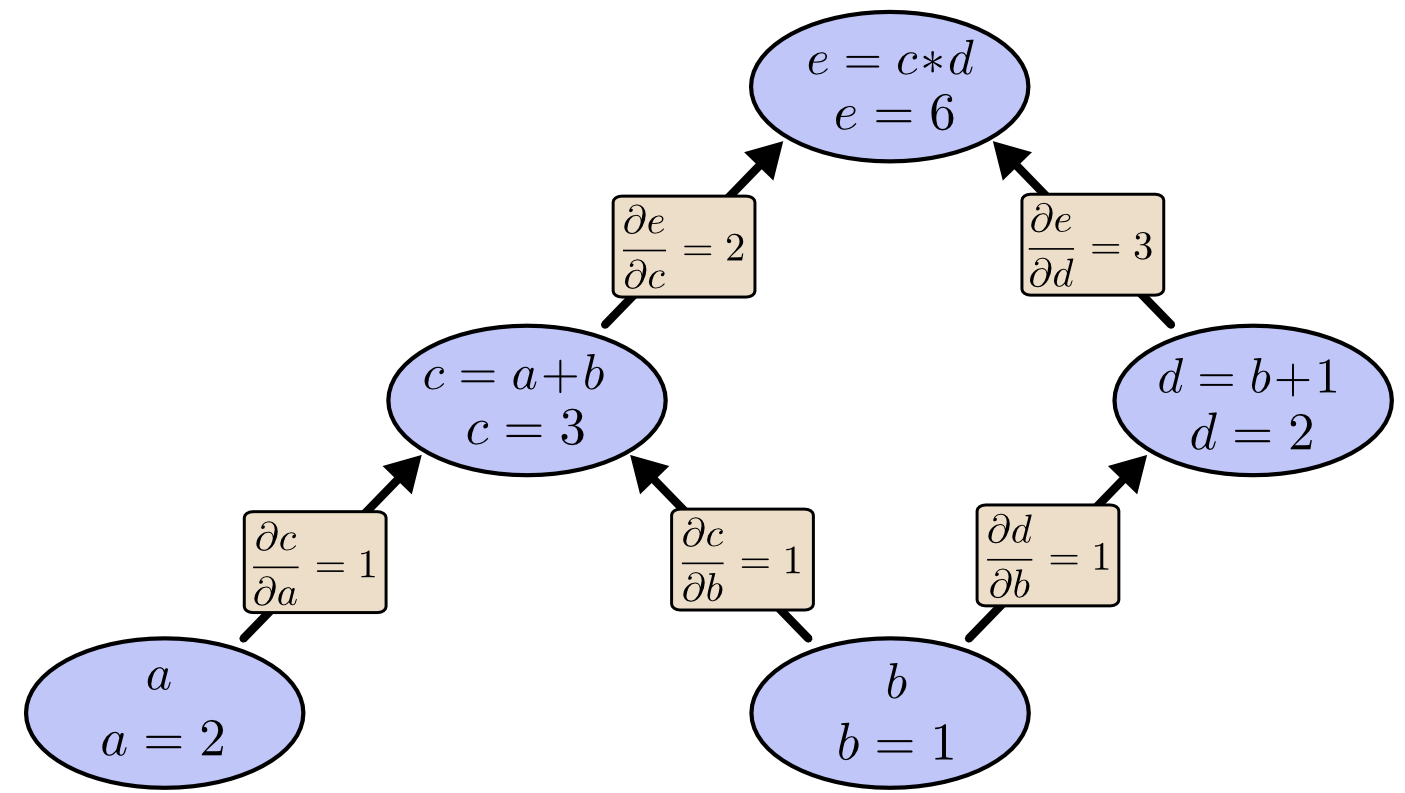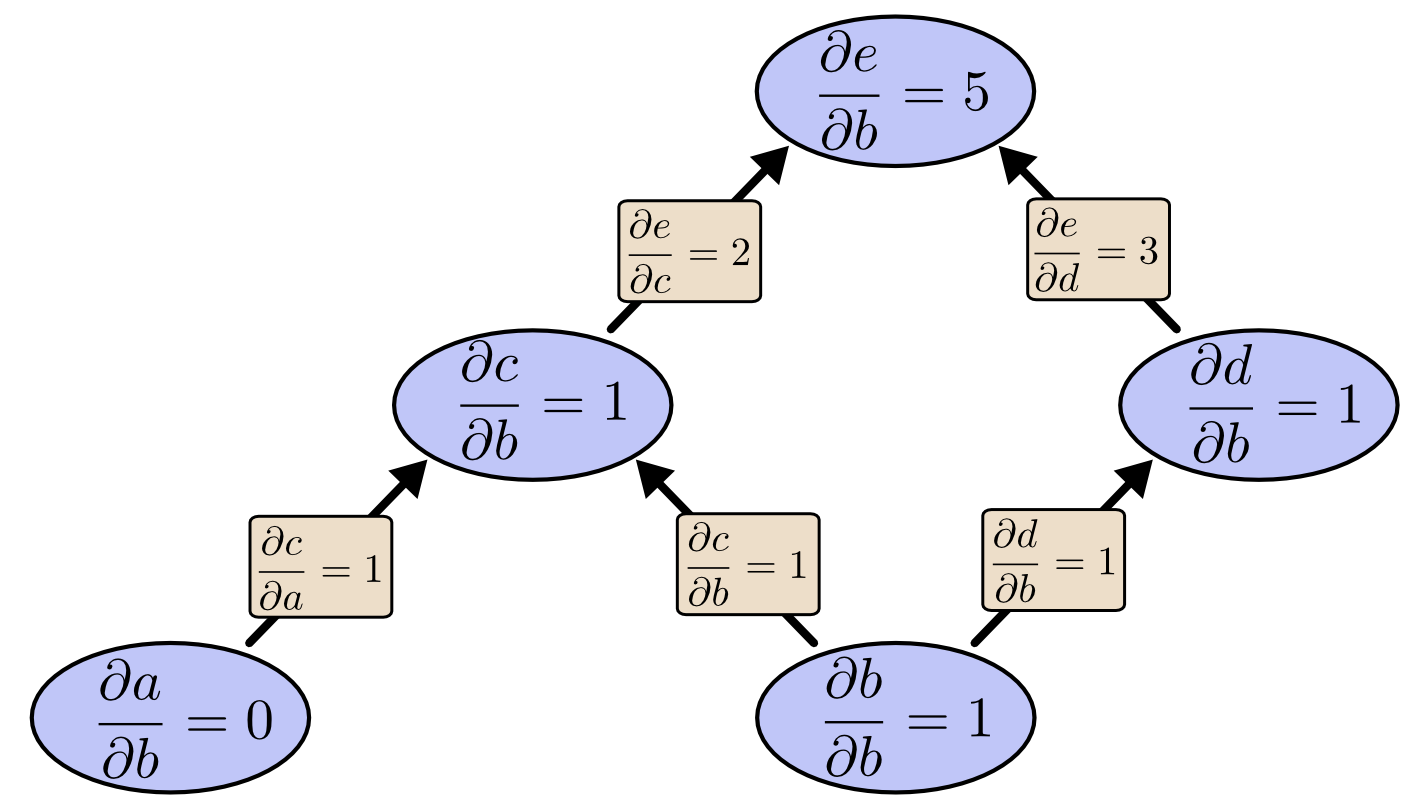# 计算图

$$c= a+b \ d=b+1 \ e=c*d$$# 计算图上的求导

$$\frac{\partial(a+b)}{\partial a} = \frac{\partial a}{\partial a}+ \frac{\partial b}{\partial a} = 1 \ \frac{\partial(uv)}{\partial u} = u \frac{\partial v}{\partial u}+ v\frac{\partial u}{\partial u} = v$$$$\frac{\partial e}{\partial b} = 12 + 13$$

# 路径分解

“路径加法” 问题会引来组合爆炸，在下面图中， $X$ 到 $Y$以及 $Y$ 到 $Z$ 中分别有3条路径，如果我们通过“路径加法”法则求 $\frac{\partial Z}{\partial X}$，我们需要计算 9 条路径：

$$\frac{\partial Z}{\partial X} = \alpha\delta + \alpha\epsilon + \alpha\zeta + \beta\delta + \beta\epsilon + \beta\zeta + \gamma\delta + \gamma\epsilon + \gamma\zeta$$$$\frac{\partial Z}{\partial X} = (\alpha + \beta + \gamma)(\delta + \epsilon + \zeta)$$# 计算的好处（有没有什么情况前向微分模式更好的情况呢？答案是肯定的。我们知道反向模式微分可以给我们一个输出相对所有输入的导数， 前向模式微分可以给我所有输出相对于一个输入的导数。如果我们有一个函数拥有非常多的输出，那么前向微分模式将变得非常非常非常地快。）

# 感谢

Reprint policy